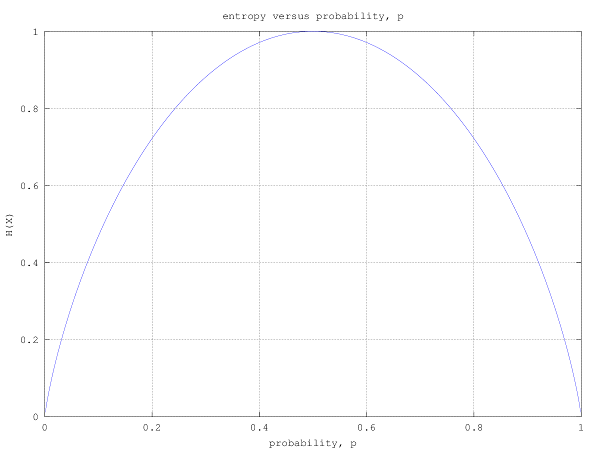- DSP log - http://www.dsplog.com -

GATE-2012 ECE Q15 (communication)

Posted By Krishna Sankar On January 25, 2013 @ 6:49 am In GATE | No Comments

Question 15 on communication from GATE (Graduate Aptitude Test in Engineering) 2012 Electronics and Communication Engineering paper.

(D) decreasesSolution

Entropy of a random variable  is defined as ,

.

Refer Chapter 2 in Elements of Information Theory, Thomas M. Cover, Joy A. Thomas (Buy from Amazon.comBuy from Flipkart.com )

Let us consider a simple case where  can take two values 1 and 0 with probability  and respectively, i.e.

.

The entropy of  is,

.

The plot of the entropy versus the probability is shown in the figure below.

clear all; close
p = [0:.001:1];
hx = -p.*log2(p) - (1-p).*log2(1-p);
plot(p,hx);
xlabel('probability, p'); ylabel('H(X)');
title('entropy versus probability, p');
axis([0 1 0 1]);grid on;Figure : Entropy versus probability for binary symmetric source

It can be see that the entropy (also termed as uncertainty) is maximum when  and for other values of , the entropy is lower. The entropy becomes 0 when  i.e. when the value of  becomes deterministic. If we extend this to a source with more than two symbols,  when probability of one of the symbols  becomes more higher than the other, the uncertainty decreases and hence entropy also decreases.

Based on the above, the right choice is (D) decreases

References

 GATE Examination Question Papers [Previous Years] from Indian Institute of Technology, Madras http://gate.iitm.ac.in/gateqps/2012/ec.pdf 

 Elements of Information Theory, Thomas M. Cover, Joy A. Thomas (Buy from Amazon.com, Buy from Flipkart.com )

URL to article: http://www.dsplog.com/2013/01/25/gate-2012-ece-q15-communication/

URLs in this post: